# Linear Regression Lines and Facets in ggplot2

In this post, we will look at how to add a regression line to a plot using the “ggplot2” package. This is mostly a review of what we learned in the post on adding a LOESS line to a plot. The main difference is that a regression line is a straight line that represents the relationship between the x and y variable while a LOESS line is used mostly to identify trends in the data.One new wrinkle we will add to this discussion is the use of faceting when developing plots. Faceting is the development of multiple plots simultaneously with each sharing different information about the data.

The data we will use is the “Housing” dataset from the “Ecdat” package. We will examine how lotsize affects housing price when also considering whether the house has central air conditioning or not. Below is the initial code in order to be prepared for analysis

``library(ggplot2);library(Ecdat)``
``````## Loading required package: Ecfun
##
## Attaching package: 'Ecdat'
##
## The following object is masked from 'package:datasets':
##
##     Orange``````
``data("Housing")``

The first plot we will make is the basic plot of lotsize and price with the data being distinguished by having central air or not, without a regression line. The code is as follows

``ggplot(data=Housing, aes(x=lotsize, y=price, col=airco))+geom_point()``We will now add the regression line to the plot. We will make a new plot with an additional piece of code.

``ggplot(data=Housing, aes(x=lotsize, y=price, col=airco))+geom_point()+stat_smooth(method='lm')``As you can see we get two lines for the two conditions of the data. If we want to see the overall regression line we use the code that is shown below.

``ggplot()+geom_point(data=Housing, aes(x=lotsize, y=price, col=airco))+stat_smooth(data=Housing, aes(x=lotsize, y=price ),method='lm')``We will now experiment with a technique called faceting. Faceting allows you to split the data by various subgroups and display the result via plot simultaneously. For example, below is the code for splitting the data by central air for examining the relationship between lot size and price.

``ggplot(data=Housing, aes(lotsize, price, col=airco))+geom_point()+stat_smooth(method='lm')+facet_grid(.~airco)``By adding the “facet_grid” function we can subset the data by the categorical variable “airco”.

In the code below we have three plots. The first two show the relationship between lotsize and price based on central air and the last plot shows the overall relationship.

``ggplot(data=Housing, aes(lotsize, price, col=airco))+geom_point()+stat_smooth(method="lm")+facet_grid(.~airco, margins = TRUE)``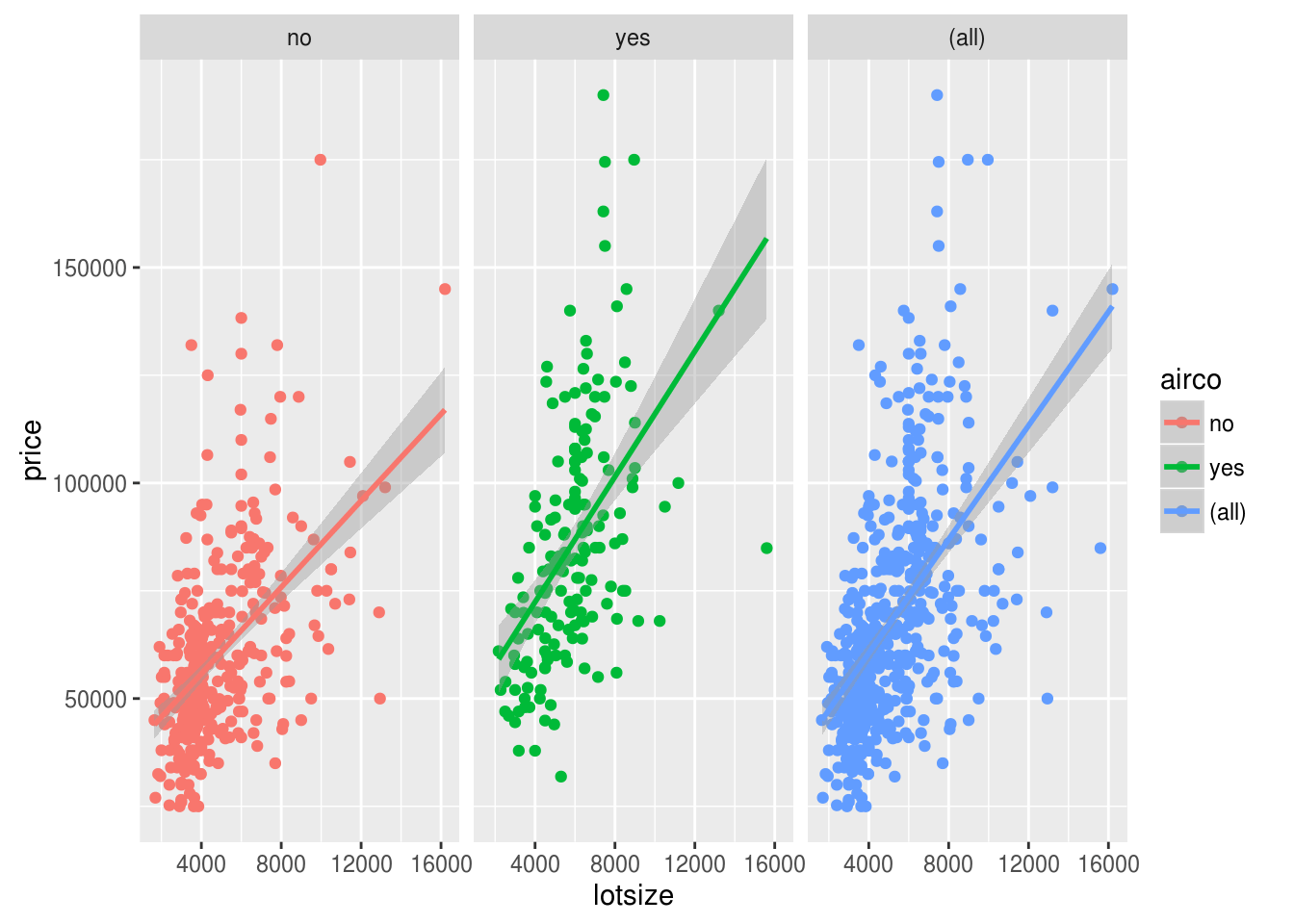By adding the argument “margins” and setting it to true we are able to add the third plot that shows the overall results.

So far all of are facetted plots have had the same statistical transformation of the use of a regression. However, we can actually mix the type of transformations that happen when facetting the results. This is shown below.

``ggplot(data=Housing, aes(lotsize, price, col=airco))+geom_point()+stat_smooth(data=subset(Housing, airco=="yes"))+stat_smooth(data=subset(Housing, airco=="no"), method="lm")+facet_grid(.~airco)``In the code we needed to use two functions of “stat_smooth” and indicate the information to transform inside the function. The plot to the left is a regression line with houses without central air and the plot to the right is a LOESS line with houses that have central air.

Conclusion

In this post, we explored the use of regression lines and advance faceting techniques. Communicating data with ggplot2 is one of many ways in which a data analyst can portray valuable information.

# Adding LOESS Lines to Plots in R

A common goal of statistics is to try and identify trends in the data as well as to predict what may happen. Both of these goals can be partially achieved through the development of graphs and or charts.In this post, we will look at adding a smooth line to a scatterplot using the “ggplot2” package.

To accomplish this, we will use the “Carseats” dataset from the “ISLR” package. We will explore the relationship between the price of carseats with actual sales along with whether the carseat was purchase in an urban location or not. Below is some initial code to prepare for the analysis.

``````library(ggplot2);library(ISLR)
data("Carseats")``````

We are going to use a layering approach in this example. This means we will add one piece of code at a time until we have the complete plot.We are now going to plot the initial scatterplot. We simply want a scatterplot depicting the relationship between Price and Sales of carseats.

``ggplot(data=Carseats, aes(x=Price, y=Sales, col=Urban))+geom_point()``The general trend appears to be negative. As price increases sales decrease regardless if carseat was purchase in an urban setting or not.

We will now add ar LOESS line to the graph. LOESS stands for “Locally weighted smoothing” this is a commonly used tool in regression analysis. The addition of a LOESS line allows in identifying trends visually much easily. Below is the code

``````ggplot(data=Carseats, aes(x=Price, y=Sales, col=Urban))+geom_point()+
stat_smooth()``````Unlike a regression line which is strictly straight, a LOESS line curves with the data. As you look at the graph the LOESS line is mostly straight with curves at the extremes and for a small rise in fall in the middle for carseats purchased in urban areas.

So far we have created LOESS lines by the categorical variable Urban. We can actually make a graph with three LOESS lines. One for Yes urban, another for No Urban, and the last one that is an overall line that does not take into account the Urban variable. Below is the code.

``ggplot()+ geom_point(data=Carseats, aes(x=Price, y=Sales, col=Urban))+ stat_smooth(data=Carseats, aes(x=Price, y=Sales))+stat_smooth(data=Carseats, aes(x=Price, y=Sales, col=Urban))``Notice that the code is slightly different with the information being mostly outside of the “ggplot” function. You can barely see the third line in the graph but if you look closely you will see a new blue line that was not there previously. This is the overall trend line. If you want you can see the overall trend line with the code below.

``ggplot()+ geom_point(data=Carseats, aes(x=Price, y=Sales, col=Urban))+ stat_smooth(data=Carseats, aes(x=Price, y=Sales))``The very first graph we generated in this post only contained points. This is because we used the “geom_point” function. Any of the graphs we created could be generated with points by removing the “geom_point” function and only using the “stat_smooth” function as shown below.

``````ggplot(data=Carseats, aes(x=Price, y=Sales, col=Urban))+
stat_smooth()``````Conclusion

This post provided an introduction to adding LOESS lines to a graph using ggplot2. For presenting data in a visually appealing way, adding lines can help in identifying key characteristics in the data.# Intro to the Grammar of Graphics

In developing graphs, there are certain core principles that need to be addressed in order to provide a graph that communicates meaning clearly to an audience. Many of these core principles are addressed in the book “The Grammar of Graphics” by Leland Wilkinson.The concepts of Wilkinson’s book were used to create the “ggplot2” r package by Hadley Wickham. This post will explain some of the core principles needed in developing high-quality visualizations. In particular, we will look at the following.

• Aesthetic attributes
• Geometric objects
• Statistical transformations
• Scales
• Coordinates
• Faceting

One important point to mention is that when using ggplot2 not all of these concepts have to be addressed in the code as R will auto-select certain features if you do not specify them.

Aesthetic Attributes and Geometric Objects

Aesthetic attributes are about how the data is perceived. This generally involves arguments in the “ggplot” relating to the x/y coordinates as well as the actual data that is being used. Aesthetic attributes are mandatory information for making a graph.

Geometric objects determine what type of plot is generated. There are many different examples such as bar, point, boxplot, and histogram.

To use the “ggplot” function you must provide the aesthetic and geometric object information to generate a plot. Below is coding containing only this information.

``````library(ggplot2)
ggplot(Orange, aes(circumference))+geom_histogram()``````
``## `stat_bin()` using `bins = 30`. Pick better value with `binwidth`.``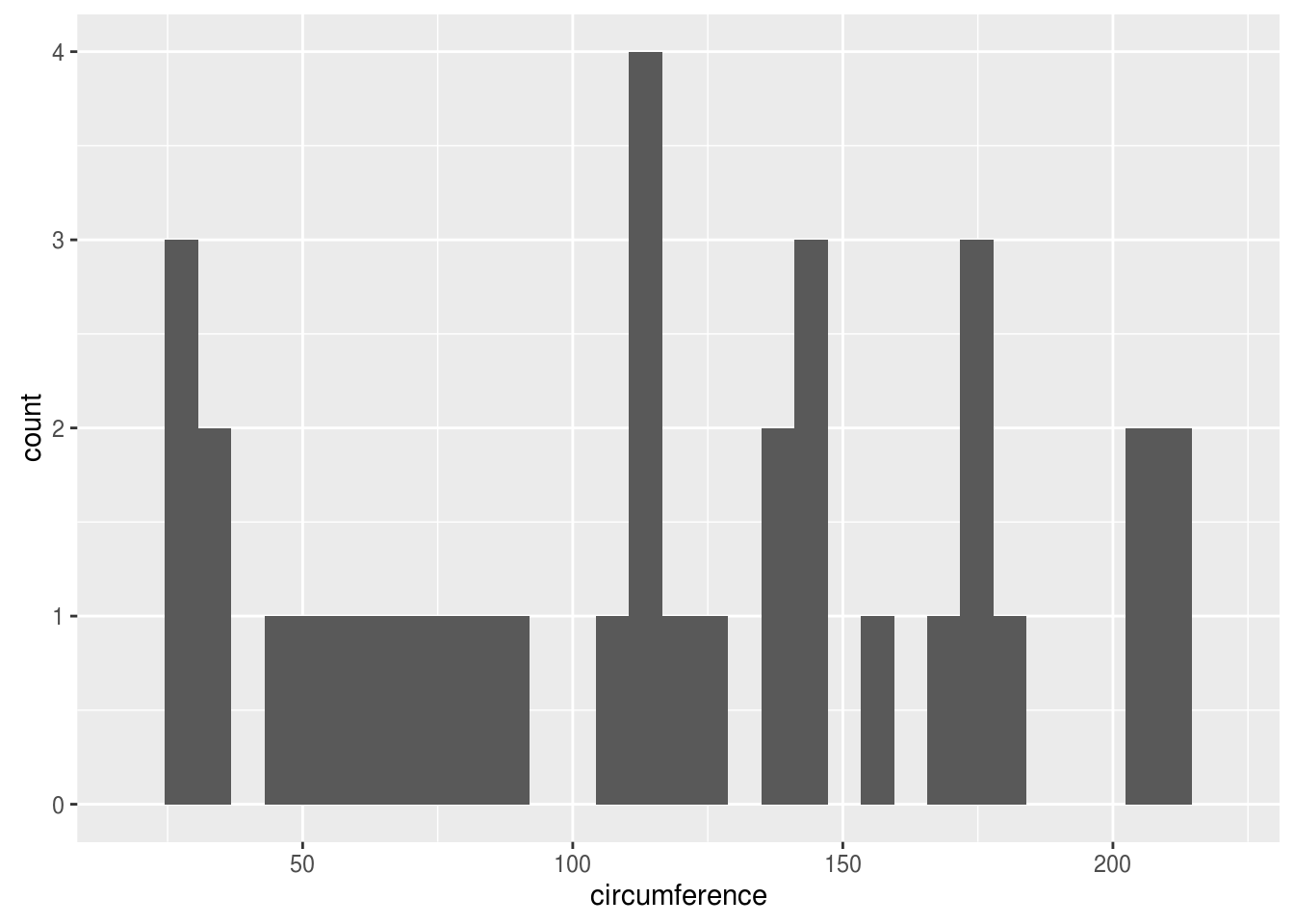The code is broken down as follows ggplot (data, aesthetic attribute(x-axis data at least)+geometric object())

Statistical Transformation Statistical transformation involves combining the data in one way or the other to get a general sense of the data. Examples of statistical transformation include adding a smooth line, a regression line, or even binning the data for histograms. This feature is optional but can provide additional explanation of the data.

Below we are going to look at two variables on one plot. For this, we will need a different geometric object as we will use points instead of a histogram. We will also use a statistical transformation. In particular, the statistical transformation is regression line. The code is as follows

``ggplot(Orange, aes(circumference, age))+geom_point()+stat_smooth(method="lm")``The code is broken down as follows ggplot (data, aesthetic attribute(x-axis data at least)+geometric object()+ statistical transformation(type of transformation))

Scales Scales is a rather complicated feature. For simplicity, scales have to do with labeling the title, x and y-axis, creating a legend, as well as the coloring of data points. This use of this feature is optional.

Below is a simple example using the “labs” function in the plot we develop in the previous example.

``ggplot(Orange, aes(circumference,age))+geom_point()+stat_smooth(method="lm") +  labs(title="Example Plot", x="circumference of the tree", y="age of the tree")``The plot now has a title and clearly labeled x and y axises

Coordinates Coordinates is another complex feature. This feature allows for the adjusting of the mapping of the data. Two common mapping features are cartesian and polar. Cartesian is commonly used for plots in 2D while polar is often used for pie charts.

In the example below, we will use the same data but this time use a polar mapping approach. The plot doesn’t make much sense but is really just an example of using this feature. This feature is also optional.

``ggplot(Orange, aes(circumference, age))+geom_point()+stat_smooth(method="lm")+labs(title="Example Plot",x="circumference of the tree", y="age of the tree")+coord_polar()``The last feature is faceting. Faceting allows you to group data in subsets. This allows you to look at your data from the perspective of various subgroups in the sample population.

In the example below, we will look at the relationship between circumference and age by tree type.

``ggplot(Orange, aes(circumference, age))+geom_point()+stat_smooth(method="lm")+labs(title="Example Plot",x="circumference of the tree", y="age of the tree")+facet_grid(Tree~.)``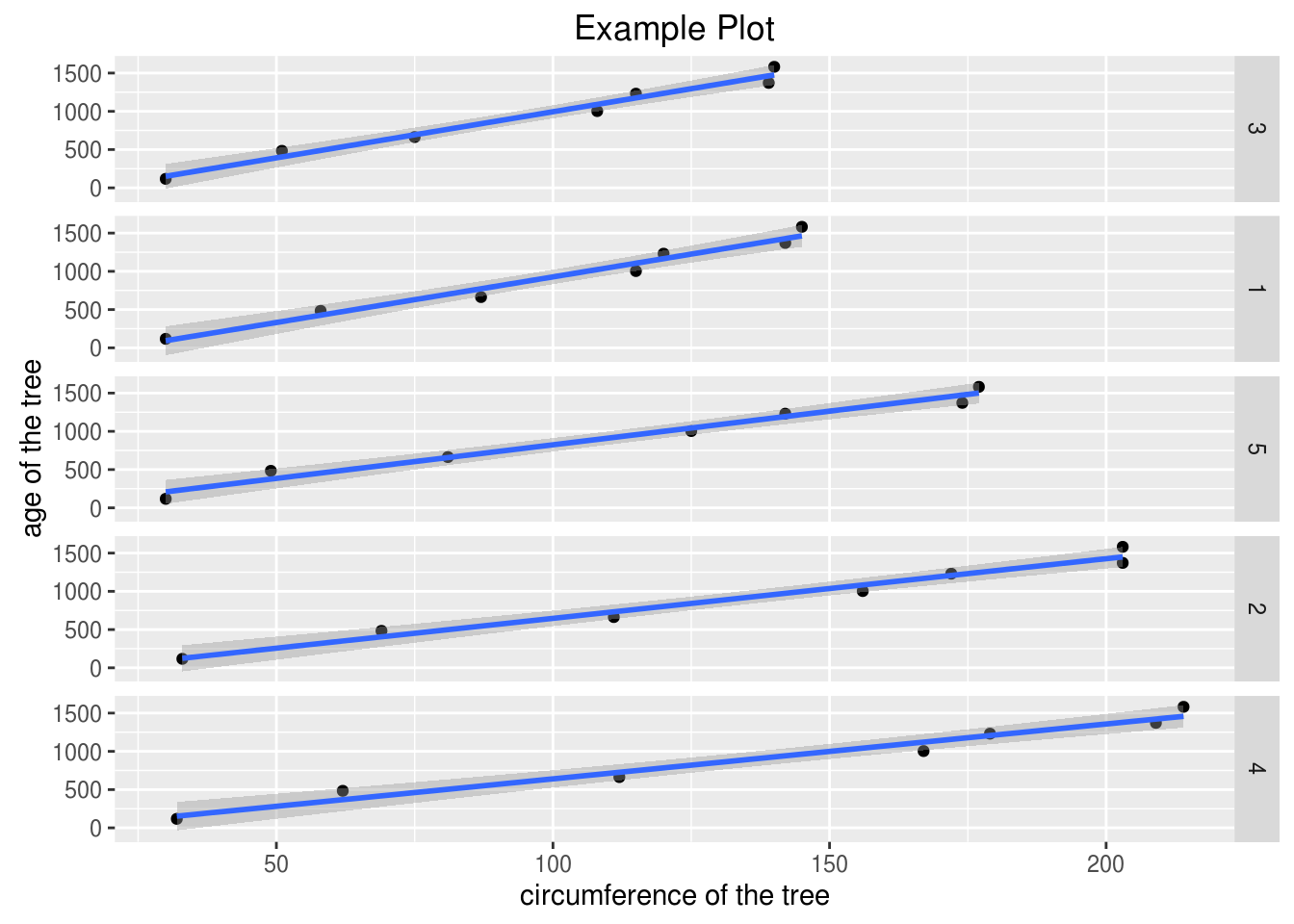Now we can see the relationship between the two variables based on the type of tree. One important thing to note about the “facet_grid” function is the use of the “.~” If this symbol “~.” is placed behind the categorical variable the charts will be stacked on top of each other is in the previous example.

However, if the symbol is written differently “.~” and placed in front of the categorical variable the plots will be placed next to each other as in the example below

``ggplot(Orange, aes(circumference, age))+geom_point()+stat_smooth(method="lm")+labs(title="Example Plot",x="circumference of the tree", y="age of the tree")+facet_grid(.~Tree)``Conclusion

This post provided an introduction to the grammar of graphics. In order to appreciate the art of data visualization, it requires understanding how the different principles of graphics work together to communicate information in a visual manner with an audience.

# Using Qplots for Graphs in R

In this post, we will explore the use of the “qplot” function from the “ggplot2” package. One of the major advantages of “ggplot” when compared to the basegraphics package in R is that the “ggplot2” plots are much more visually appealing. This will make more sense when we explore the grammar of graphics. for now, we will just make plots to get used to using the “qplot” function.

We are going to use the “Carseats” dataset from the “ISLR” package in the examples. This dataset has data about the purchase of carseats for babies. Below is the initial code you will need to make the various plots.

``````library(ggplot2);library(ISLR)
data("Carseats")``````

In the first scatterplot, we are going to compare the price of a carseat with the volume of sales. Below is the code

``qplot(Price, Sales,data=Carseats)``Most of this coding format you are familiar. “Price” is the x variable. “Sales” is the y variable and the data used is “Carseats. From the plot, we can see that as the price of the carseat increases there is normally a decline in the number of sales.

For our next plot, we will compare sales based on shelf location. This requires the use of a boxplot. Below is the code

``qplot(ShelveLoc, Sales, data=Carseats, geom="boxplot")``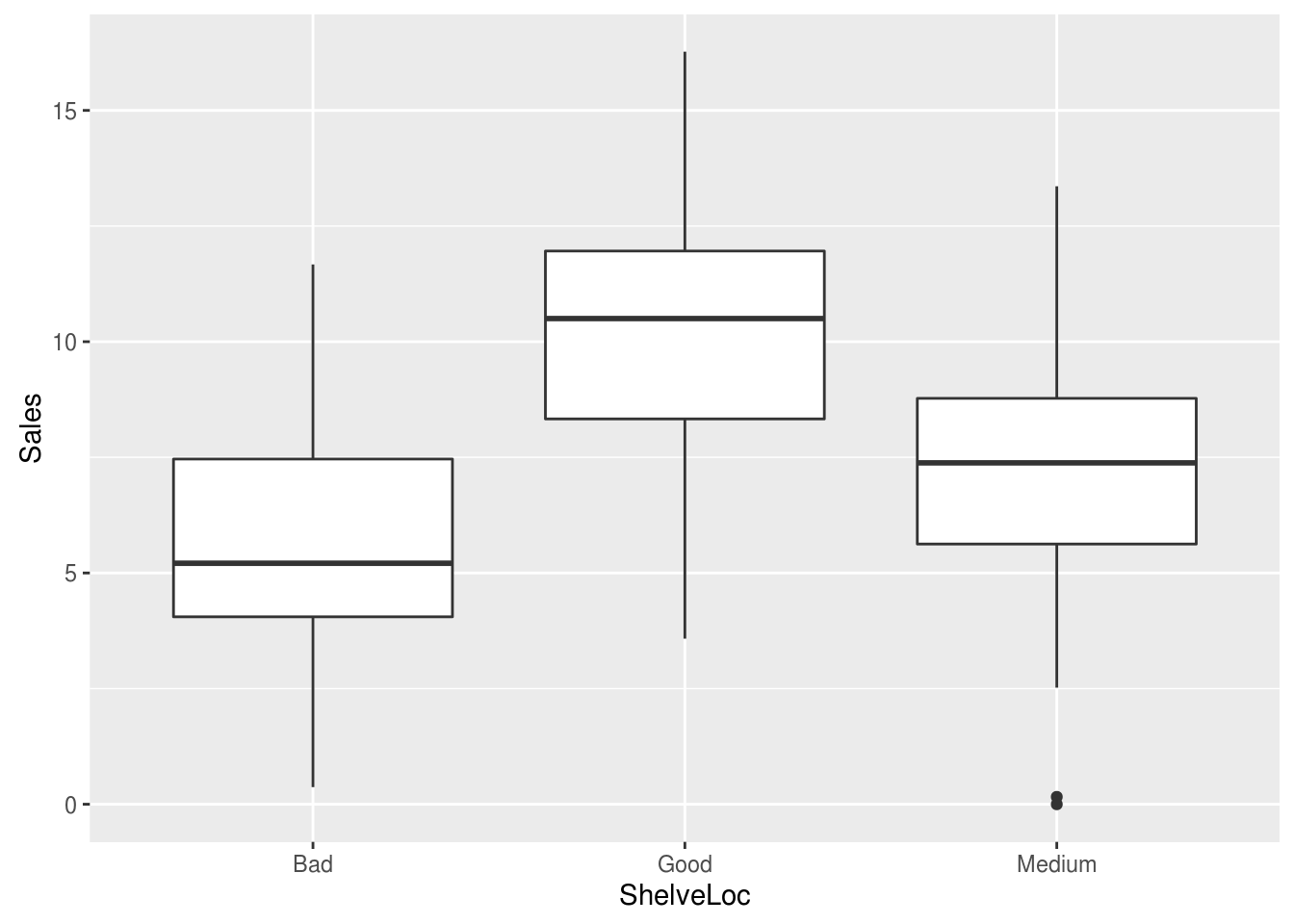The new argument in the code is the “geom” argument. This argument indicates what type of plot is drawn.

The boxplot appears to indicate that a “good” shelf location has the best sales. However, this would need to be confirmed with a statistical test.

Perhaps you are wondering how many of the Carseats were in the bad, medium, and good shelf locations. To find out, we will make a barplot as shown in the code below

``qplot(ShelveLoc, data=Carseats, geom="bar")``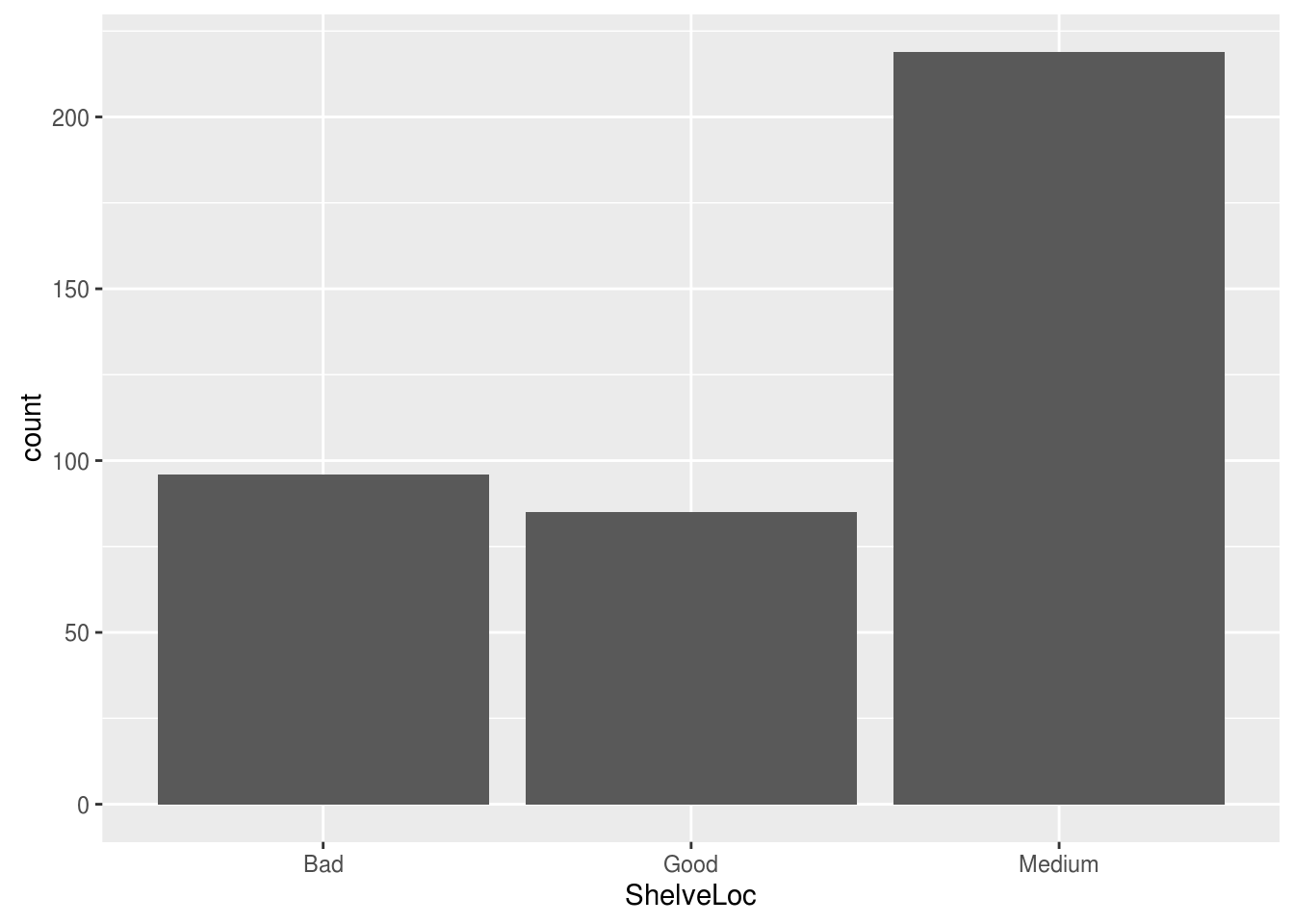The most common location was medium with bad and good be almost equal.

Lastly, we will now create a histogram using the “qplot” function. We want to see the distribution of “Sales”. Below is the code

``qplot(Sales, data=Carseats, geom="histogram")``
``## `stat_bin()` using `bins = 30`. Pick better value with `binwidth`.``The distribution appears to be normal but again to know for certain requires a statistical test. For one last, trick we will add the median to the plot by using the following code

``qplot(Sales, data=Carseats, geom="histogram") + geom_vline(xintercept = median(Carseats\$Sales), colour="blue")``
``## `stat_bin()` using `bins = 30`. Pick better value with `binwidth`.``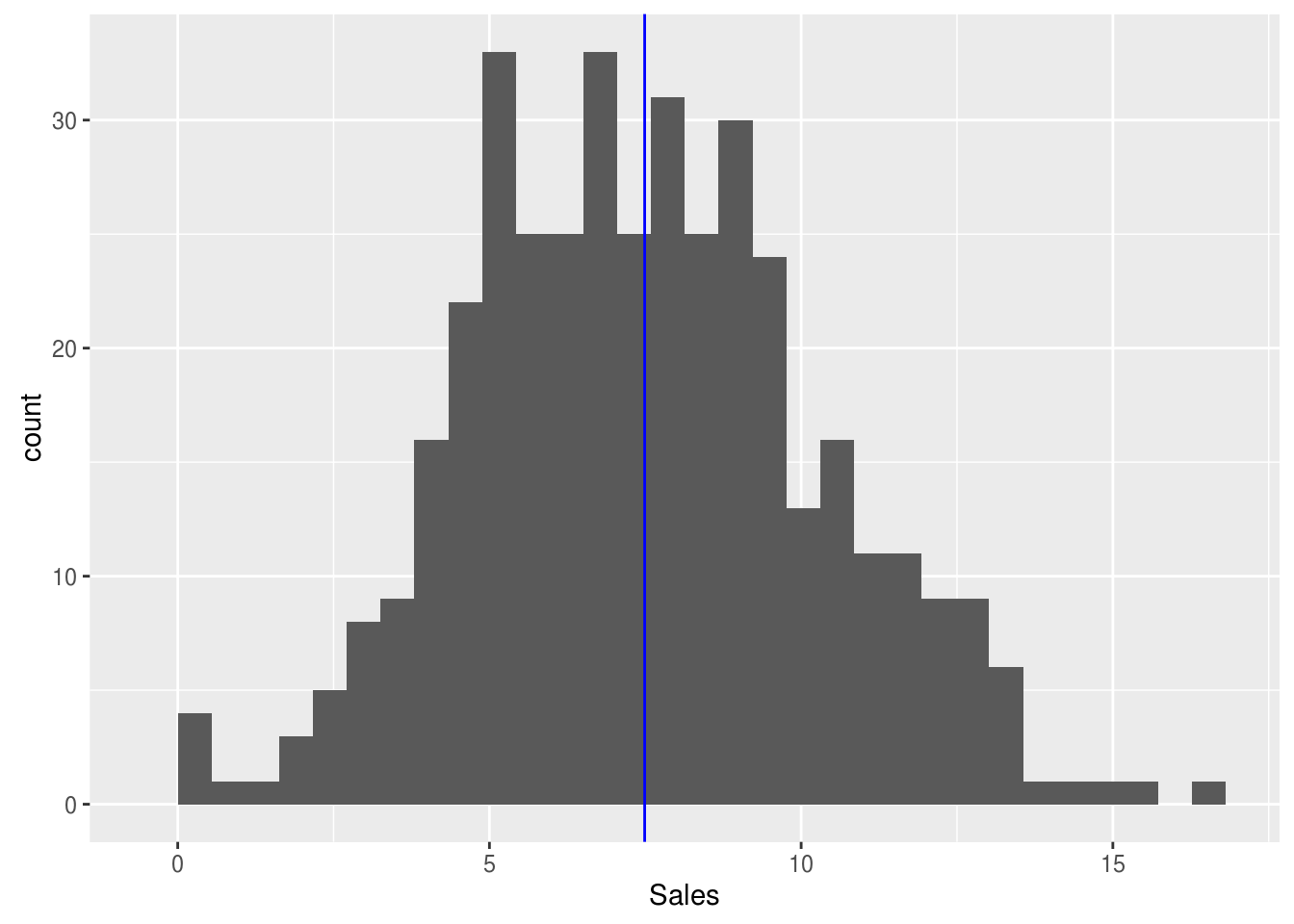To add the median all we needed to do was add an additional argument called “geom_vline” which adds a line to a plot. Inside this argument, we had to indicate what to add by indicating the median of “Sales” from the “Carseats” package.

Conclusion

This post provided an introduction to the use of the “qplot” function in the “ggplot2” package. Understanding the basics of “qplor” is beneficial in providing visually appealing graphics

# Making Graphics in R

Data visualization is a critical component of communicate results with an audience. Fortunately, R provides many different ways to present numerical data it a clear way visually. This post will look specifically at making data visualizations with the base r package “graphics”.

Generally, functions available in the “graphics” package can be either high-level functions or low-level functions. High-level functions actually make the plot. Examples of high-level functions includes are the “hist” (histogram), “boxplot” (boxplot), and “barplot” (bar plot).

Low-level functions are used to add additional information to a plot. Some commonly used low-level functions includes “legend” (add legend) and “text” (add text). When coding we allows call high-level functions before low-level functions as the other way is not accepted by R.

We are going to begin with a simple graph. We are going to use the“Caschool” dataset from the “Ecdat” package. For now, we are going to plot the average expenditures per student by the average number of computers per student. Keep in mind that we are only plotting the data so we are only using a high-level function (plot). Below is the code

``library(Ecdat)``
``````data("Caschool")
plot(compstu~expnstu, data=Caschool)``````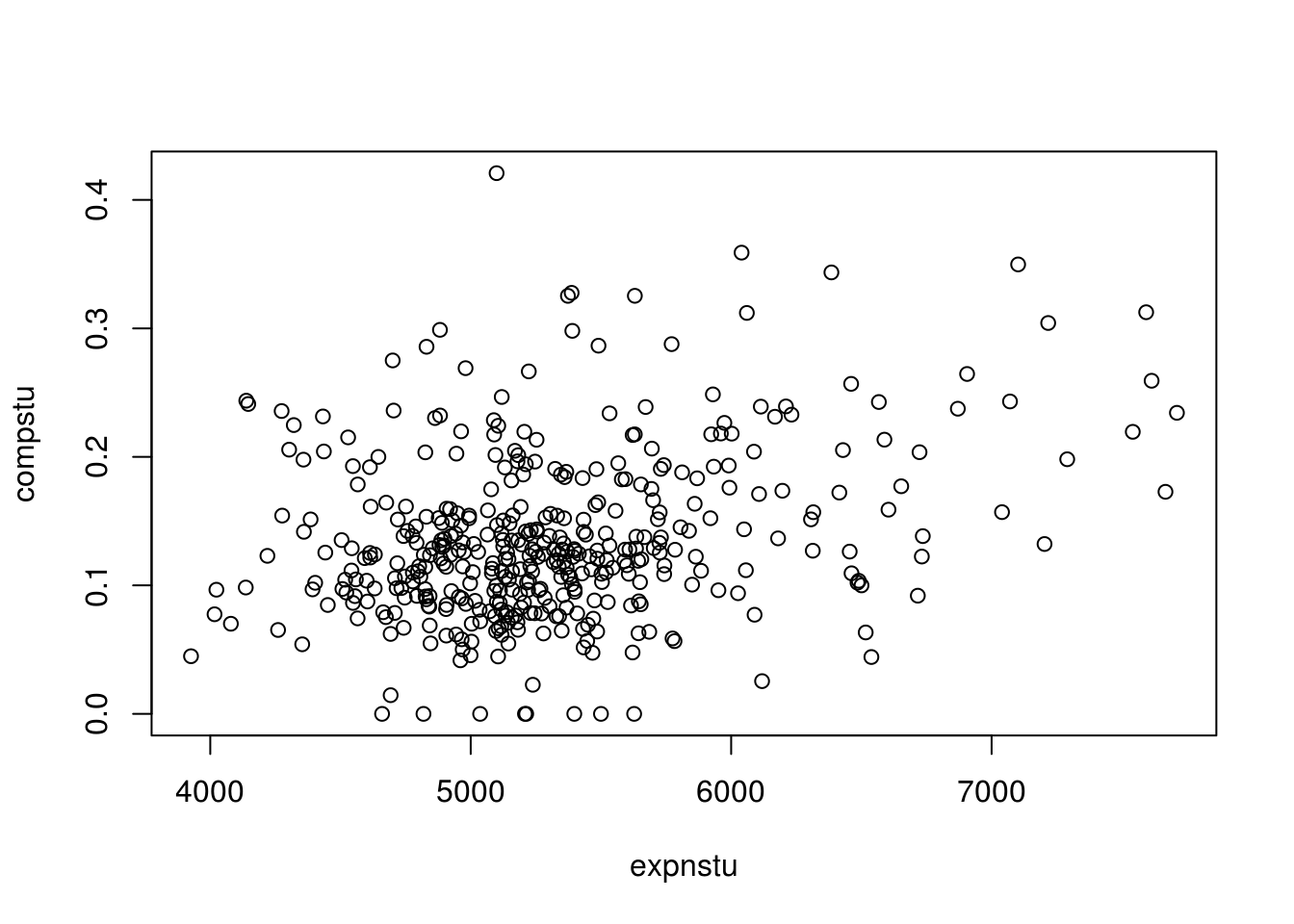The plot is not “beautiful” but it is a start in plotting data. Next, we are going to add a low-level function to our code. In particular, we will add a regression line to try and see the diretion of the relationship between the two variables via a straight line. In addition, we will use the “loess.smooth” function. This function will allow us to see the general shape of the data. The regression line is green and the loess smooth line is blue. The coding is mostly familiy but the “lwd” argument allows us to make the line thicker.

``````plot(compstu~expnstu, data=Caschool)
abline(lm(compstu~expnstu, data=Caschool), col="green", lwd=5)
lines(loess.smooth(Caschool\$expnstu, Caschool\$compstu), col="blue", lwd=5)``````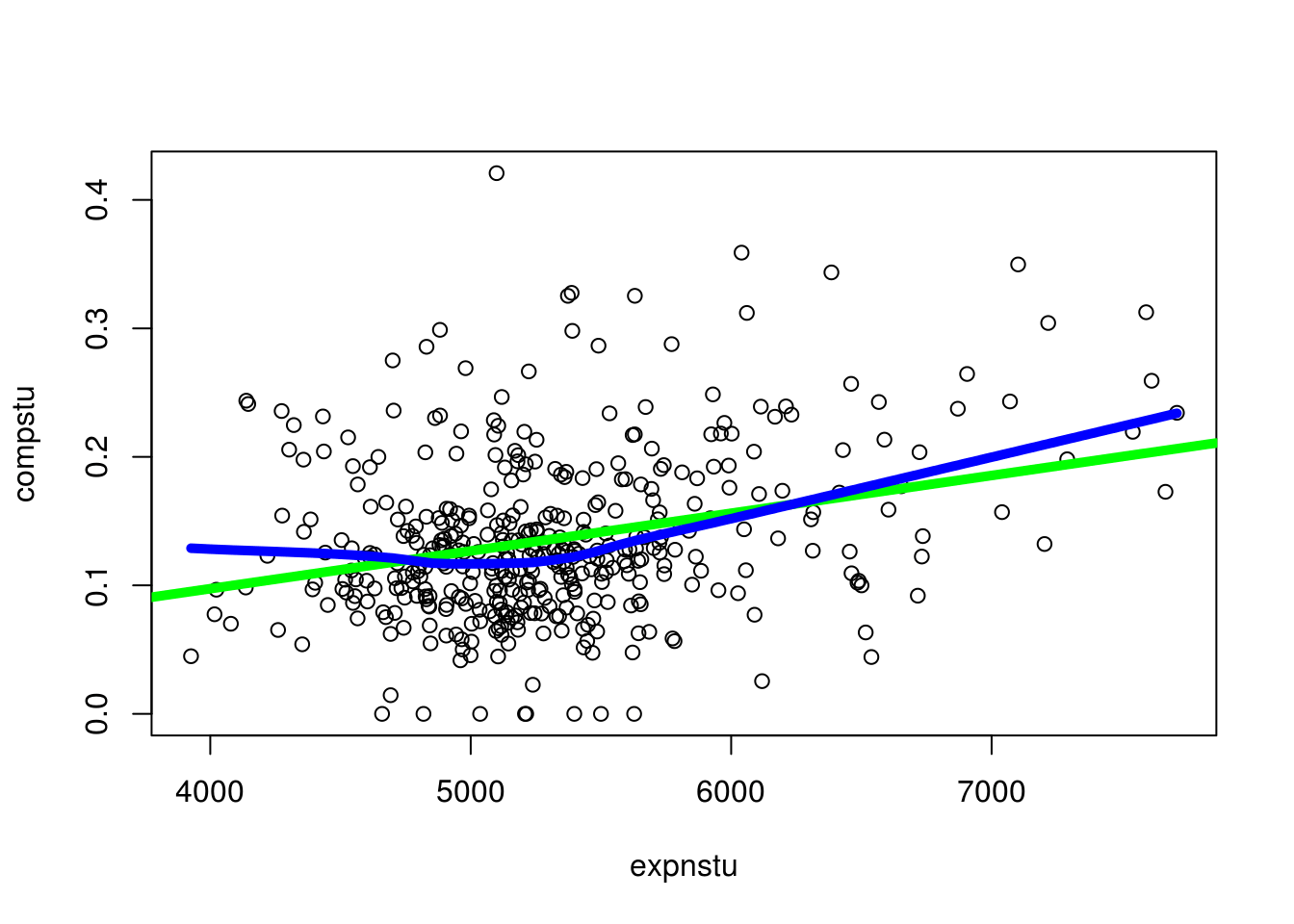Boxplots allow you to show data that has been subsetted in some way. This allows for the comparisions of groups. In addition, one or more boxplots can be used to identify outliers.

In the plot below, the student-to-teacher ratio of k-6 and k-8 grades are displayed.

``boxplot(str~grspan, data=Caschool)``As you look at the data you can see there is very little difference. However, one major differnce is that the K-8 group has much more extreme values than K-6.

Histograms are an excellent way to display information about one continuous variable. In the plot below, we can see the spread of the expenditure per student.

``hist(Caschool\$expnstu)``We will now add median to the plot by calling the low-level function “abline”. Below is the code.

``````hist(Caschool\$expnstu)
abline(v=median(Caschool\$expnstu), col="green", lwd=5)``````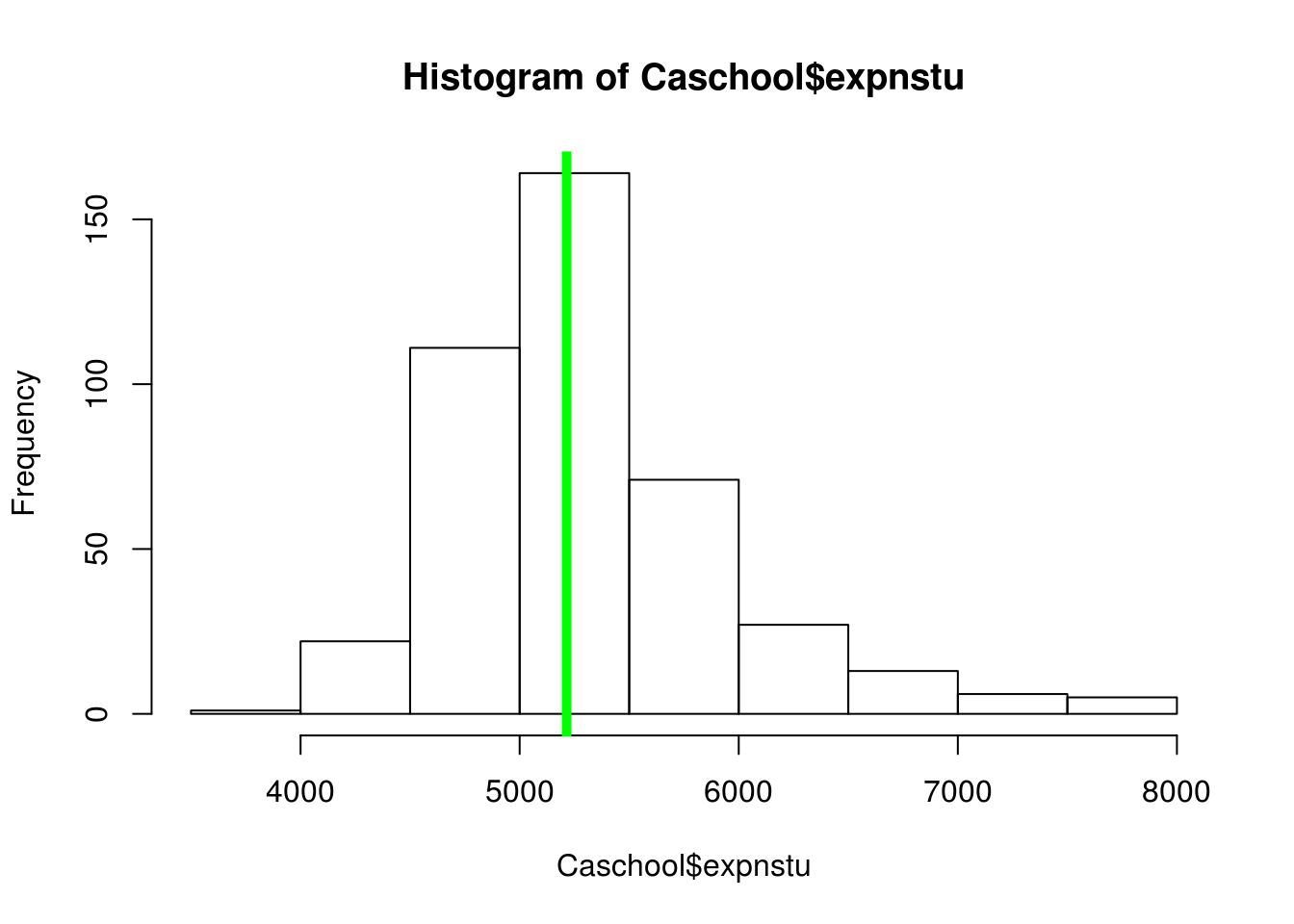Conclusion

In this post, we learned some of the basic structures of creating plots using the “graphics” package. All plots in include both low and high-level functions that work together to draw and provide additional information for communicating data in a visual manner

# Logistic Regression in R

Logistic regression is used when the dependent variable is categorical with two choices. For example, if we want to predict whether someone will default on their loan. The dependent variable is categorical with two choices yes they default and no they do not.Interpreting the output of a logistic regression analysis can be tricky. Basically, you need to interpret the odds ratio. For example, if the results of a study say the odds of default are 40% higher when someone is unemployed it is an increase in the likelihood of something happening. This is different from the probability which is what we normally use. Odds can go from any value from negative infinity to positive infinity. Probability is constrained to be anywhere from 0-100%.

We will now take a look at a simple example of logistic regression in R. We want to calculate the odds of defaulting on a loan. The dependent variable is “default” which can be either yes or no. The independent variables are “student” which can be yes or no, “income” which how much the person made, and “balance” which is the amount remaining on their credit card.

Below is the coding for developing this model.

The first step is to load the “Default” dataset. This dataset is a part of the “ISLR” package. Below is the code to get started

``````library(ISLR)
data("Default")``````

It is always good to examine the data first before developing a model. We do this by using the ‘summary’ function as shown below.

``summary(Default)``
``````##  default    student       balance           income
##  No :9667   No :7056   Min.   :   0.0   Min.   :  772
##  Yes: 333   Yes:2944   1st Qu.: 481.7   1st Qu.:21340
##                        Median : 823.6   Median :34553
##                        Mean   : 835.4   Mean   :33517
##                        3rd Qu.:1166.3   3rd Qu.:43808
##                        Max.   :2654.3   Max.   :73554``````

We now need to check our two continuous variables “balance” and “income” to see if they are normally distributed. Below is the code followed by the histograms.

``hist(Default\$income)``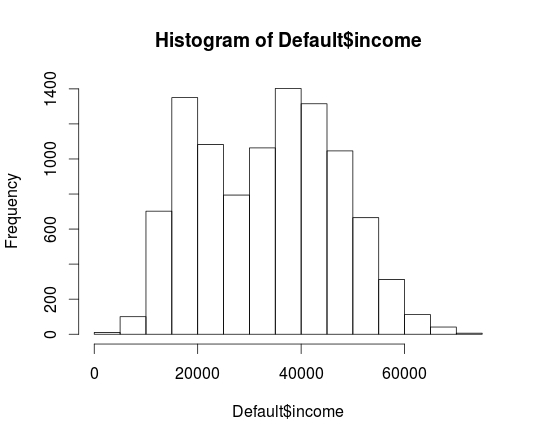``hist(Default\$balance)``The ‘income’ variable looks fine but there appear to be some problems with ‘balance’ to deal with this we will perform a square root transformation on the ‘balance’ variable and then examine it again by looking at a histogram. Below is the code.

``````Default\$sqrt_balance<-(sqrt(Default\$balance))
hist(Default\$sqrt_balance)``````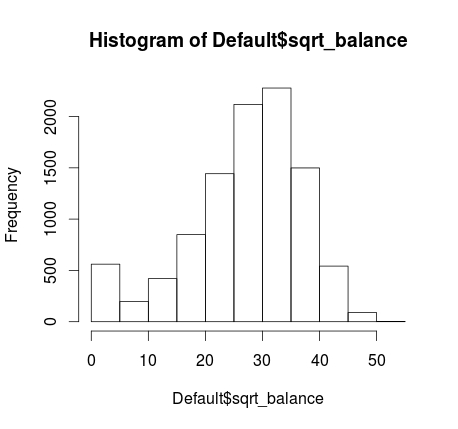As you can see this is much better looking.

We are now ready to make our model and examine the results. Below is the code.

``````Credit_Model<-glm(default~student+sqrt_balance+income, family=binomial, Default)
summary(Credit_Model)``````
``````##
## Call:
## glm(formula = default ~ student + sqrt_balance + income, family = binomial,
##     data = Default)
##
## Deviance Residuals:
##     Min       1Q   Median       3Q      Max
## -2.2656  -0.1367  -0.0418  -0.0085   3.9730
##
## Coefficients:
##                Estimate Std. Error z value Pr(>|z|)
## (Intercept)  -1.938e+01  8.116e-01 -23.883  < 2e-16 ***
## studentYes   -6.045e-01  2.336e-01  -2.587  0.00967 **
## sqrt_balance  4.438e-01  1.883e-02  23.567  < 2e-16 ***
## income        3.412e-06  8.147e-06   0.419  0.67538
## ---
## Signif. codes:  0 '***' 0.001 '**' 0.01 '*' 0.05 '.' 0.1 ' ' 1
##
## (Dispersion parameter for binomial family taken to be 1)
##
##     Null deviance: 2920.6  on 9999  degrees of freedom
## Residual deviance: 1574.8  on 9996  degrees of freedom
## AIC: 1582.8
##
## Number of Fisher Scoring iterations: 9``````

The results indicate that the variable ‘student’ and ‘sqrt_balance’ are significant. However, ‘income’ is not significant. What all this means in simple terms is that being a student and having a balance on your credit card influence the odds of going into default while your income makes no difference. Unlike, multiple regression coefficients, the logistic coefficients require a transformation in order to interpret them The statistical reason for this is somewhat complicated. As such, below is the code to interpret the logistic regression coefficients.

``exp(coef(Credit_Model))``
``````##  (Intercept)   studentYes sqrt_balance       income
## 3.814998e-09 5.463400e-01 1.558568e+00 1.000003e+00``````

To explain this as simply as possible. You subtract 1 from each coefficient to determine the actual odds. For example, if a person is a student the odds of them defaulting are 445% higher than when somebody is not a student when controlling for balance and income. Furthermore, for every 1 unit increase in the square root of the balance the odds of default go up by 55% when controlling for being a student and income. Naturally, speaking in terms of a 1 unit increase in the square root of anything is confusing. However, we had to transform the variable in order to improve normality.

Conclusion

Logistic regression is one approach for predicting and modeling that involves a categorical dependent variable. Although the details are little confusing this approach is valuable at times when doing an analysis.

# Assumption Check for Multiple Regression

The goal of the post is to attempt to explain the salary of a baseball based on several variables. We will see how to test various assumptions of multipleregression as well as deal with missing data. The first thing we need to do is load our data. Our data will come from the “ISLR” package and we will use the dataset “Hitters”. There are 20 variables in the dataset as shown by the “str” function

``````#Load data
library(ISLR)
data("Hitters")
str(Hitters)``````
``````## 'data.frame':    322 obs. of  20 variables:
##  \$ AtBat    : int  293 315 479 496 321 594 185 298 323 401 ...
##  \$ Hits     : int  66 81 130 141 87 169 37 73 81 92 ...
##  \$ HmRun    : int  1 7 18 20 10 4 1 0 6 17 ...
##  \$ Runs     : int  30 24 66 65 39 74 23 24 26 49 ...
##  \$ RBI      : int  29 38 72 78 42 51 8 24 32 66 ...
##  \$ Walks    : int  14 39 76 37 30 35 21 7 8 65 ...
##  \$ Years    : int  1 14 3 11 2 11 2 3 2 13 ...
##  \$ CAtBat   : int  293 3449 1624 5628 396 4408 214 509 341 5206 ...
##  \$ CHits    : int  66 835 457 1575 101 1133 42 108 86 1332 ...
##  \$ CHmRun   : int  1 69 63 225 12 19 1 0 6 253 ...
##  \$ CRuns    : int  30 321 224 828 48 501 30 41 32 784 ...
##  \$ CRBI     : int  29 414 266 838 46 336 9 37 34 890 ...
##  \$ CWalks   : int  14 375 263 354 33 194 24 12 8 866 ...
##  \$ League   : Factor w/ 2 levels "A","N": 1 2 1 2 2 1 2 1 2 1 ...
##  \$ Division : Factor w/ 2 levels "E","W": 1 2 2 1 1 2 1 2 2 1 ...
##  \$ PutOuts  : int  446 632 880 200 805 282 76 121 143 0 ...
##  \$ Assists  : int  33 43 82 11 40 421 127 283 290 0 ...
##  \$ Errors   : int  20 10 14 3 4 25 7 9 19 0 ...
##  \$ Salary   : num  NA 475 480 500 91.5 750 70 100 75 1100 ...
##  \$ NewLeague: Factor w/ 2 levels "A","N": 1 2 1 2 2 1 1 1 2 1 ...``````

We now need to assess the amount of missing data. This is important because missing data can cause major problems with the different analysis. We are going to create a simple function that will explain to us the amount of missing data for each variable in the “Hitters” dataset. After using the function we need to use the “apply” function to display the results according to the amount of data missing by column and row.

``````Missing_Data <- function(x){sum(is.na(x))/length(x)*100}
apply(Hitters,2,Missing_Data)``````
``````##     AtBat      Hits     HmRun      Runs       RBI     Walks     Years
##   0.00000   0.00000   0.00000   0.00000   0.00000   0.00000   0.00000
##    CAtBat     CHits    CHmRun     CRuns      CRBI    CWalks    League
##   0.00000   0.00000   0.00000   0.00000   0.00000   0.00000   0.00000
##  Division   PutOuts   Assists    Errors    Salary NewLeague
##   0.00000   0.00000   0.00000   0.00000  18.32298   0.00000``````
apply(Hitters,1,Missing_Data)``````

For column, we can see that the missing data is all in the salary variable, which is missing 18% of its data. By row (not displayed here) you can see that a row might be missing anywhere from 0-5% of its data. The 5% is from the fact that there are 20 variables and there is only missing data in the salary variable. Therefore 1/20 = 5% missing data for a row. To deal with the missing data, we will use the ‘mice’ package. You can install it yourself and run the following code

``library(mice)``
``md.pattern(Hitters)``
``````##     AtBat Hits HmRun Runs RBI Walks Years CAtBat CHits CHmRun CRuns CRBI
## 263     1    1     1    1   1     1     1      1     1      1     1    1
##  59     1    1     1    1   1     1     1      1     1      1     1    1
##         0    0     0    0   0     0     0      0     0      0     0    0
##     CWalks League Division PutOuts Assists Errors NewLeague Salary
## 263      1      1        1       1       1      1         1      1  0
##  59      1      1        1       1       1      1         1      0  1
##          0      0        0       0       0      0         0     59 59``````
``Hitters1 <- mice(Hitters,m=5,maxit=50,meth='pmm',seed=500)``
``summary(Hitters1)``
``````## Multiply imputed data set
## Call:
## mice(data = Hitters, m = 5, method = "pmm", maxit = 50, seed = 500)

``````

In the code above we did the following

1. loaded the ‘mice’ package Run the ‘md.pattern’ function Made a new variable called ‘Hitters’ and ran the ‘mice’ function on it.
2. This function made 5 datasets  (m = 5) and used predictive meaning matching to guess the missing data point for each row (method = ‘pmm’).
3. The seed is set for the purpose of reproducing the results The md.pattern function indicates that

There are 263 complete cases and 59 incomplete ones (not displayed). All the missing data is in the ‘Salary’ variable. The ‘mice’ function shares various information of how the missing data was dealt with. The ‘mice’ function makes five guesses for each missing data point. You can view the guesses for each row by the name of the baseball player. We will then select the first dataset as are new dataset to continue the analysis using the ‘complete’ function from the ‘mice’ package.

``````#View Imputed data
Hitters1\$imp\$Salary``````
``````#Make Complete Dataset
completedData <- complete(Hitters1,1)``````

Now we need to deal with the normality of each variable which is the first assumption we will deal with. To save time, I will only explain how I dealt with the non-normal variables. The two variables that were non-normal were “salary” and “Years”. To fix these two variables I did a log transformation of the data. The new variables are called ‘log_Salary’ and “log_Years”. Below is the code for this with the before and after histograms

``````#Histogram of Salary
hist(completedData\$Salary)``````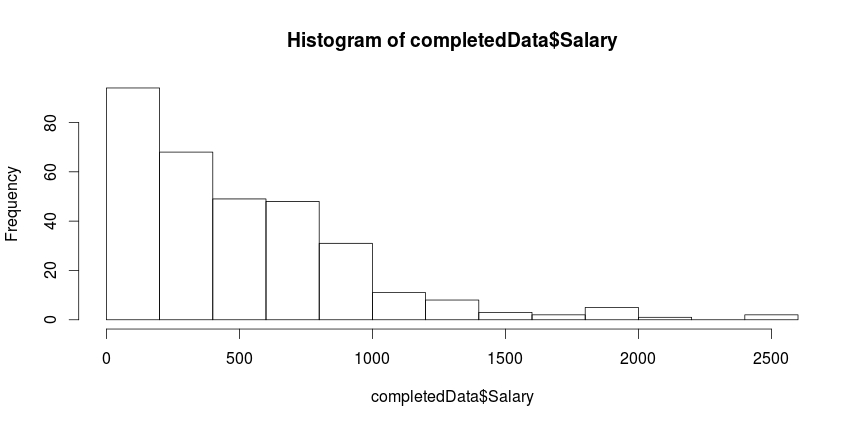``````#log transformation of Salary
completedData\$log_Salary<-log(completedData\$Salary)
#Histogram of transformed salary
hist(completedData\$log_Salary)`````````#Histogram of years
hist(completedData\$Years)```
``````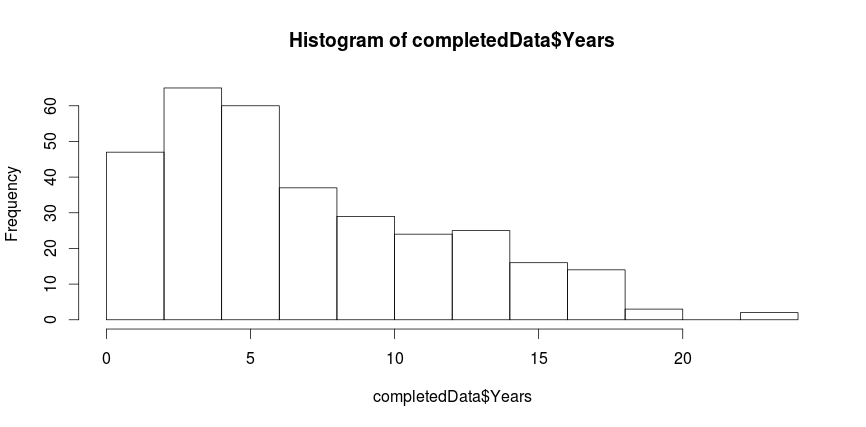#Log transformation of Years completedData\$log_Years<-log(completedData\$Years) hist(completedData\$log_Years)``````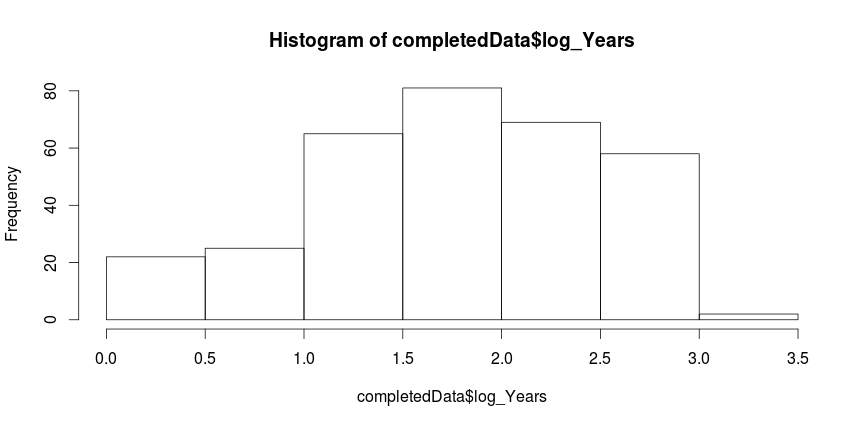We can now do are regression analysis and produce the residual plot in order to deal with the assumption of homoscedasticity and linearity. Below is the code

``````Salary_Model<-lm(log_Salary~Hits+HmRun+Walks+log_Years+League, data=completedData)
#Residual Plot checks Linearity
plot(Salary_Model)``````

When using the ‘plot’ function you will get several plots. The first is the residual vs fitted which assesses linearity. The next is the qq plot which explains if our data is normally distributed. The scale location plot explains if there is equal variance. The residual vs leverage plot is used for finding outliers. All plots look good.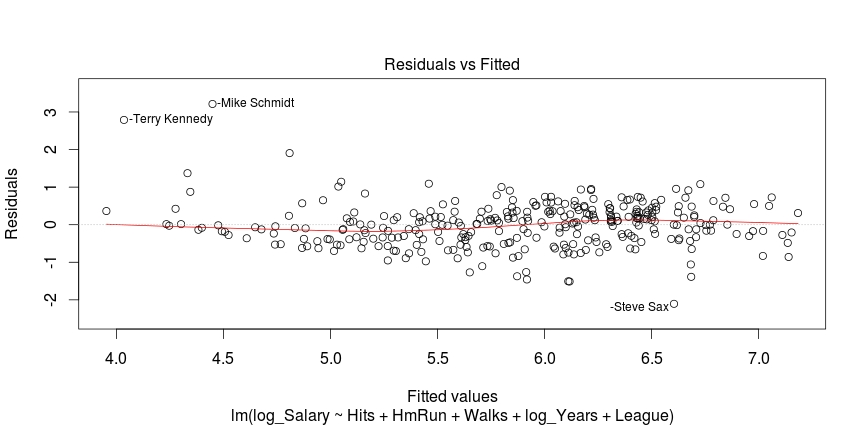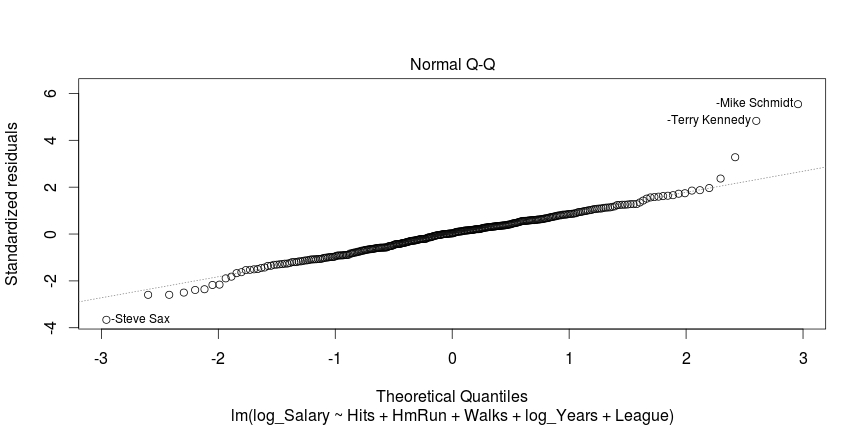``summary(Salary_Model)``
``````##
## Call:
## lm(formula = log_Salary ~ Hits + HmRun + Walks + log_Years +
##     League, data = completedData)
##
## Residuals:
##     Min      1Q  Median      3Q     Max
## -2.1052 -0.3649  0.0171  0.3429  3.2139
##
## Coefficients:
##              Estimate Std. Error t value Pr(>|t|)
## (Intercept) 3.8790683  0.1098027  35.328  < 2e-16 ***
## Hits        0.0049427  0.0009928   4.979 1.05e-06 ***
## HmRun       0.0081890  0.0046938   1.745  0.08202 .
## Walks       0.0063070  0.0020284   3.109  0.00205 **
## log_Years   0.6390014  0.0429482  14.878  < 2e-16 ***
## League2     0.1217445  0.0668753   1.820  0.06963 .
## ---
## Signif. codes:  0 '***' 0.001 '**' 0.01 '*' 0.05 '.' 0.1 ' ' 1
##
## Residual standard error: 0.5869 on 316 degrees of freedom
## Multiple R-squared:  0.5704, Adjusted R-squared:  0.5636
## F-statistic: 83.91 on 5 and 316 DF,  p-value: < 2.2e-16``````

Furthermore, the model explains 57% of the variance in salary. All variables (Hits, HmRun, Walks, Years, and League) are significant at 0.1. Our last step is to find the correlations among the variables. To do this, we need to make a correlational matrix. We need to remove variables that are not a part of our study to do this. We also need to load the “Hmisc” package and use the ‘rcorr’ function to produce the matrix along with the p values. Below is the code

``````#find correlation
completedData1<-completedData;completedData1\$Chits<-NULL;completedData1\$CAtBat<-NULL;completedData1\$CHmRun<-NULL;completedData1\$CRuns<-NULL;completedData1\$CRBI<-NULL;completedData1\$CWalks<-NULL;completedData1\$League<-NULL;completedData1\$Division<-NULL;completedData1\$PutOuts<-NULL;completedData1\$Assists<-NULL; completedData1\$NewLeague<-NULL;completedData1\$AtBat<-NULL;completedData1\$Runs<-NULL;completedData1\$RBI<-NULL;completedData1\$Errors<-NULL; completedData1\$CHits<-NULL;completedData1\$Years<-NULL; completedData1\$Salary<-NULL
library(Hmisc)``````
`` rcorr(as.matrix(completedData1))``
``````##            Hits HmRun Walks log_Salary log_Years
## Hits       1.00  0.56  0.64       0.47      0.13
## HmRun      0.56  1.00  0.48       0.36      0.14
## Walks      0.64  0.48  1.00       0.46      0.18
## log_Salary 0.47  0.36  0.46       1.00      0.63
## log_Years  0.13  0.14  0.18       0.63      1.00
##
## n= 322
##
##
## P
##            Hits   HmRun  Walks  log_Salary log_Years
## Hits              0.0000 0.0000 0.0000     0.0227
## HmRun      0.0000        0.0000 0.0000     0.0153
## Walks      0.0000 0.0000        0.0000     0.0009
## log_Salary 0.0000 0.0000 0.0000            0.0000
## log_Years  0.0227 0.0153 0.0009 0.0000``````

There are no high correlations among our variables so multicollinearity is not an issue

Conclusion

This post provided an example dealing with missing data, checking the assumptions of a regression model, and displaying plots. All this was done using R.

# Decision Trees in R

Decision trees are useful for splitting data based into smaller distinct groups based on criteria you establish. This post will attempt to explain how to develop decision trees in R.We are going to use the ‘College’ dataset found in the “ISLR” package. Once you load the package you need to split the data into a training and testing set as shown in the code below. We want to divide the data based on education level, age, and income

```library(ISLR); library(ggplot2); library(caret)
data("College")
inTrain<-createDataPartition(y=College\$education,
p=0.7, list=FALSE)
trainingset <- College[inTrain, ]
testingset <- College[-inTrain, ]```

Visualize the Data

We will now make a plot of the data based on education as the groups and age and wage as the x and y variable. Below is the code followed by the plot. Please note that education is divided into 5 groups as indicated in the chart.

```qplot(age, wage, colour=education, data=trainingset)Create the Model```

We are now going to develop the model for the decision tree. We will use age and wage to predict education as shown in the code below.

`TreeModel<-train(education~age+income, method='rpart', data=trainingset)`

Create Visual of the Model

We now need to create a visual of the model. This involves installing the package called ‘rattle’. You can install ‘rattle’ separately yourself. After doing this below is the code for the tree model followed by the diagram.

`fancyRpartPlot(TreeModel\$finalModel)`Here is what the chart means

1. At the top is node 1 which is called ‘HS Grad” the decimals underneath is the percentage of the data that falls within the “HS Grad” category. As the highest node, everything is classified as “HS grad” until we begin to apply our criteria.
2. Underneath nod 1 is a decision about wage. If a person makes less than 112 you go to the left if they make more you go to the right.
3. Nod 2 indicates the percentage of the sample that was classified as HS grade regardless of education. 14% of those with less than a HS diploma were classified as a HS Grade based on wage. 43% of those with a HS diploma were classified as a HS grade based on income. The percentage underneath the decimals indicates the total amount of the sample placed in the HS grad category. Which was 57%.
4. This process is repeated for each node until the data is divided as much as possible.

Predict

You can predict individual values in the dataset by using the ‘predict’ function with the test data as shown in the code below.

predict(TreeModel, newdata = testingset)

Conclusion

Prediction Trees are a unique feature in data analysis for determining how well data can be divided into subsets. It also provides a visual of how to move through data sequentially based on characteristics in the data.

# Using Plots for Prediction in R

It is common in machine learning to look at the training set of your data visually. This helps you to decide what to do as you begin to build your model.  In this post, we will make several different visual representations of data using datasets available in several R packages.

We are going to explore data in the “College” dataset in the “ISLR” package. If you have not done so already, you need to download the “ISLR” package along with“ggplot2” and the “caret” package.

Once these packages are installed in R you want to look at a summary of the variables use the summary function as shown below.

`summary(College)`

You should get a printout of information about 18 different variables. Based on this printout, we want to explore the relationship between graduation rate “Grad.Rate” and student to faculty ratio “S.F.Ratio”. This is the objective of this post.

Next, we need to create a training and testing dataset below is the code to do this.

```> library(ISLR);library(ggplot2);library(caret)
> data("College")
> PracticeSet<-createDataPartition(y=College\$Enroll, p=0.7, +                                  list=FALSE) > trainingSet<-College[PracticeSet,] > testSet<-College[-PracticeSet,] > dim(trainingSet); dim(testSet)
 545  18
 232  18```

The explanation behind this code was covered in predicting with caret so we will not explain it again. You just need to know that the dataset you will use for the rest of this post is called “trainingSet”.

Developing a Plot

We now want to explore the relationship between graduation rates and student to faculty ratio. We will be used the ‘ggpolt2’  package to do this. Below is the code for this followed by the plot.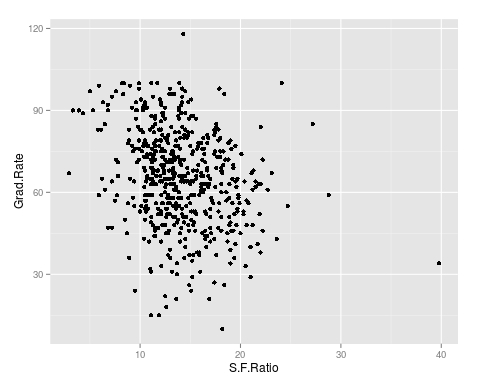As you can see, there appears to be a negative relationship between student faculty ratio and grad rate. In other words, as the ration of student to faculty increases there is a decrease in the graduation rate.

Next, we will color the plots on the graph based on whether they are a public or private university to get a better understanding of the data. Below is the code for this followed by the plot.

> qplot(S.F.Ratio, Grad.Rate, colour = Private, data=trainingSet)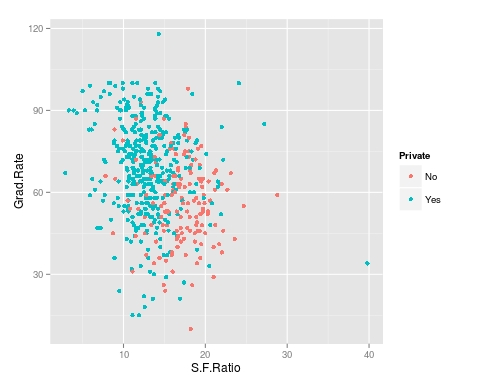It appears that private colleges usually have lower student to faculty ratios and also higher graduation rates than public colleges

We will now plot the same data but will add a regression line. This will provide us with a visual of the slope. Below is the code followed by the plot.

> collegeplot<-qplot(S.F.Ratio, Grad.Rate, colour = Private, data=trainingSet) > collegeplot+geom_smooth(method = ‘lm’,formula=y~x)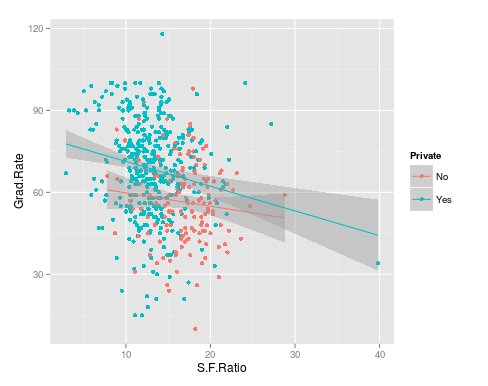Most of this code should be familiar to you. We saved the plot as the variable ‘collegeplot’. In the second line of code, we add specific coding for ‘ggplot2’ to add the regression line. ‘lm’ means linear model and formula is for creating the regression.

Cutting the Data

We will now divide the data based on the student-faculty ratio into three equal size groups to look for additional trends. To do this you need the “Hmisc” packaged. Below is the code followed by the table

```> library(Hmisc)
```
```> divide_College<-cut2(trainingSet\$S.F.Ratio, g=3)
> table(divide_College)
divide_College
[ 2.9,12.3) [12.3,15.2) [15.2,39.8]
185         179         181```

Our data is now divided into three equal sizes.

Box Plots

Lastly, we will make a box plot with our three equal size groups based on student-faculty ratio. Below is the code followed by the box plot

CollegeBP<-qplot(divide_College, Grad.Rate, data=trainingSet, fill=divide_College, geom=c(“boxplot”)) > CollegeBP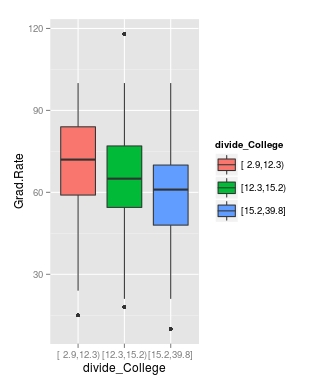As you can see, the negative relationship continues even when student-faculty is divided into three equally size groups. However, our information about private and public college is missing. To fix this we need to make a table as shown in the code below.

```> CollegeTable<-table(divide_College, trainingSet\$Private)
> CollegeTable

divide_College  No Yes
[ 2.9,12.3)  14 171
[12.3,15.2)  27 152
[15.2,39.8] 106  75```

This table tells you how many public and private colleges there based on the division of the student-faculty ratio into three groups. We can also get proportions by using the following

```> prop.table(CollegeTable, 1)

divide_College         No        Yes
[ 2.9,12.3) 0.07567568 0.92432432
[12.3,15.2) 0.15083799 0.84916201
[15.2,39.8] 0.58563536 0.41436464```

In this post, we found that there is a negative relationship between student-faculty ratio and graduation rate. We also found that private colleges have a lower student-faculty ratio and a higher graduation rate than public colleges. In other words, the status of a university as public or private moderates the relationship between student-faculty ratio and graduation rate.

You can probably tell by now that R can be a lot of fun with some basic knowledge of coding.

# Intro to Making Plots in R

One of the strongest points of R in the opinion of many are the various features for creating graphs and other visualizations of data. In this post, we begin to look at using the various visualization features of R. Specifically, we are going to do the following

• Using data in R to display graph
• Add text to a graph
• Manipulate the appearance of data in a graph

Using Plots

The ‘plot’ function is one of the basic options for graphing data. We are goingto go through an example using the ‘islands’ data that comes with the R software. The ‘islands’ software includes lots of data, in particular, it contains data on the lass mass of different islands. We want to plot the land mass of the seven largest islands. Below is the code for doing this.

`islandgraph<-head(sort(islands, decreasing=TRUE), 7)`
`plot(islandgraph, main = "Land Area", ylab = "Square Miles")`
`text(islandgraph, labels=names(islandgraph), adj=c(0.5,1))`

Here is what we did

1. We made the variable ‘islandgraph’
2. In the variable ‘islandgraph’ We used the ‘head’ and the ‘sort’ function. The sort function told R to sort the ‘island’ data by decreasing value ( this is why we have the decreasing argument equaling TRUE). After sorting the data, the ‘head’ function tells R to only take the first 7 values of ‘island’ (see the 7 in the code) after they are sorted by decreasing order.
3. Next, we use the plot function to plot are information in the ‘islandgraph’ variable. We also give the graph a title using the ‘main’ argument followed by the title. Following the title, we label the y-axis using the ‘ylab’ argument and putting in quotes “Square Miles”.
4. The last step is to add text to the information inside the graph for clarity. Using the ‘text’ function, we tell R to add text to the ‘islandgraph’ variable using the names from the ‘islandgraph’ data which uses the code ‘label=names(islandgraph)’. Remember the ‘islandgraph’ data is the first 7 islands from the ‘islands’ dataset.
5. After telling R to use the names from the islandgraph dataset we then tell it to place the label a little of center for readability reasons with the code ‘adj = c(0.5,1).

Below is what the graph should look like.Changing Point Color and Shape in a Graph

For visual purposes, it may be beneficial to manipulate the color and appearance of several data points in a graph. To do this, we are going to use the ‘faithful’ dataset in R. The ‘faithful’ dataset indicates the length of eruption time and how long people had to wait for the eruption. The first thing we want to do is plot the data using the “plot” function.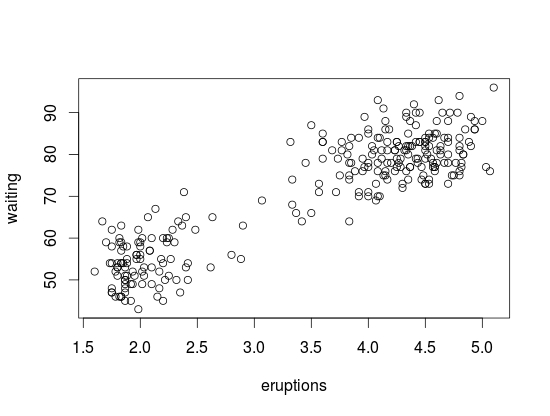As you see the data, there are two clear clusters. One contains data from 1.5-3 and the second cluster contains data from 3.5-5. To help people to see this distinction we are going to change the color and shape of the data points in the 1.5-3 range. Below is the code for this.

`eruption_time<-with(faithful, faithful[eruptions < 3, ])`
`plot(faithful)`
`points(eruption_time, col = "blue", pch = 24)`

Here is what we did

1. We created a variable named ‘eruption_time’
2. In this variable, we use the ‘with’ function. This allows us to access columns in the dataframe without having to use the \$ sign constantly. We are telling R to look at the ‘faithful’ dataframe and only take the information from faithful that has eruptions that are less than 3. All of this is indicated in the first line of code above.
3. Next, we plot ‘faithful’ again
4. Last, we add the points from are ‘eruption_time’ variable and we tell R to color these points blue and to use a different point shape by using the ‘pch = 24’ argument
5. The results are belowConclusion

In this post, we learned the following

• How to make a graph
• How to add a title and label the y-axis
• How to change the color and shape of the data points in a graph